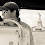## January 15, 2011

### How to sum up the values in a file

Once you know how to remove a column from a file, then a time will come where you'll want to sum up the values in a column. For this example, let's take a look at a file with numbers in it.

```mike@shiner \$ cat file-with-numbers
1
2
3
4
5
6
```

We can sum up the values in this file with the following command.

```mike@shiner \$ cat file-with-numbers | awk '{sum+=\$1}END{print sum}'
21
```

We can see here that the sum of the numbers in the file total to 21.

1.# In ruby:
sum = 0
File.open('numbers').each { |line| sum += line.to_i }
puts sum

Any more difficult than your example and I'd certainly fall back on a scripting language.

2.I agree. Is there a way to run that from the command line? Your example made me think of the following perl one-liner:

cat file-with-numbers | perl -ne '\$n += \$_; print \$n if eof'

3.ruby -e "sum=0;File.open('numbers').each { |line| sum += line.to_i };puts sum"

Run from the same directory the file is in.

4.Or, chaining together a bunch of method calls to do this in one statement:

ruby -e "puts File.open('numbers').read.split.inject(0) {|result, element| result + element.to_i}"

But this starts to get a bit crazy. But is it worse than your whacky perl '\$' variables?

5.After some playing i think i got a more cryptic ruby version...

6.Otto,
Hah..nice. I had no idea you could call eval on a string like that and have it work. That language surprises me daily still.

7.I really like the pure awk solution. Simple. Tweakable (for files that have the num in a different column) and succinct. Nice work. Don't need the cat though ;)

awk '{n+=\$1}END{print n}' file-with-numbers

8.I can't let otto always one up me with ruby so here the ganky php solution.

cat numfile | php -R '@\$c+=\$argn;' -E 'print(\$c);'

9.This one is pretty pure. No programming languages involved.

echo `cat file-with-numbers` | tr ' ' '+' | bc